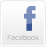HOMEMY TEACHING TOOLSFORUMABOUT USCONTACT USHome > Self-Study Quizzes > QuizGMAT Math practice - (5/36 questions - random)GMAT practice questions.  Once you complete the quiz you will see your score and explanation of the correct answer.
1.  For what values of 'k' will the pair of equations 3x + 4y = 12 and kx + 12y = 30 not have a unique solution?

 A) 12 B) 9 C) 3 D) 7.5 E) 2.5

2.  How many diagonals does a 63-sided convex polygon have?

 A) 3780 B) 1890 C) 3843 D) 3906 E) 1953

3.  If both 112 and 33 are factors of the number a * 43 * 62 * 1311, then what is the smallest possible value of a?

 A)  121 B)  3267 C)  363 D)  33 E)  None of the above

4.  If 10, 12 and 'x' are sides of an acute angled triangle, how many integer values of 'x' are possible?

 7 12 9 13 11

5.  If the mean of numbers 28, x, 42, 78 and 104 is 62, then what is the mean of 128, 255, 511, 1023 and x?

 A) 395 B) 275 C) 355 D) 415 E) 365STUDENT ACTIVITIES WEBTOOLS CUSTOMER SERVICE COMPANY INFORMATION